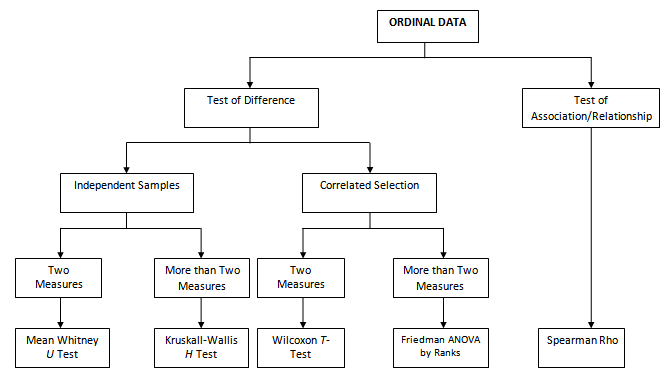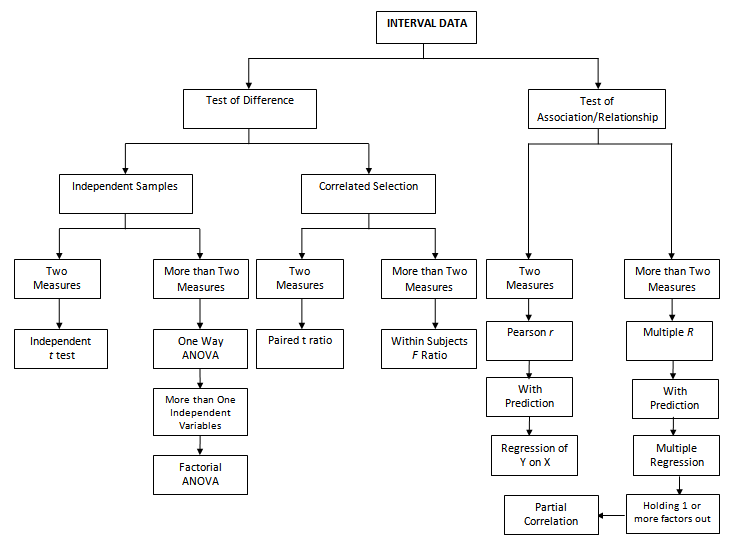## Thursday, September 26, 2019

### Level of Measurement and the Application of Data Analysis

Choosing the appropriate statistical treatment will depend on the kind of data involved in the study. The level of measurement is the process of observing and recording observations that are collected as a part of a research effort. The type of data may be nominal, ordinal, or interval. You need to have a knowledge of what type of data you have in order to apply the appropriate statistical test.

1.     Nominal or categorical data is the basic level of measurement. Examples of nominal data are gender, blood type, religion, marital status, young or old. The nominal data uses numbers to label discrete, but it does not imply order. The number or code is used for the purpose of a label or description.  The numeric codes assigned in nominal data do not convey information. If we classify male as 1 and female as 2, the numbers have no inherent meaning. The number 2 clearly does not mean ‘more than 1’. The numbers are merely symbols that represent two different values of the gender attribute.
Nominal data must have categories that are mutually exclusive and collectively exhaustive. For example, if we are to obtain ethnicity, we may use the following codes:
1 = American
2 = African-American
3 = Hispanic
4 = Asian
To present a nominal data you may use pie-chart, bar chart, or column chart. Moreover, to summarize, we use Frequency/Percentage. We cannot use Mean or Average in this type of measurement. The numbers used in nominal data cannot be treated mathematically. For example, calculating the average race of a sample. We can, however, count the elements in the categories based on the frequency of occurrences. For example, in a sample of 50 employees, we can say that 5 out of 50 or 10% are Asian, 15 out of 50 or 30% are Hispanic, 20 out of 50 or 40% are American, and 10 out of 50 or 20% are African-American. No further mathematical operations would be meaningful with the nominal level of measurement.

2.     Ordinal data involves sorting the objects on the basis of their relative standing on an attribute. The attributes are ordered according to some criterion.  If a researcher rank-orders subjects from heaviest to lightest, ordinal measurement has been used. Other examples are:
Rank in a class test (first, second or third)
Customer satisfaction ratings (On a scale of 0-10)
Socio-economic status
Education qualification
Unlike nominal data, ordinal data's information concerns not only equivalence but relative standing among objects is involved. For example, this scheme is for coding a client’s ability to perform daily activities:
(1) Completely dependent
(2) Needs  assistance
(3) Completely independent
In this case, the order is ordinal. The numbers are not arbitrary- they signify incremental ability to perform daily activities. Individuals assigned a value of three is equivalent to each other with regard to functional ability. Ordinal data provide information regarding the greater than or less than status, but not how much greater or lesser. We do not know if being completely independent is twice as good as need assistance. Nor do we know if the difference between needing assistance is the same as that of being completely independent. Ordinal level of measurement tells us only the relative ranking of the attribute’s levels.
To present ordinal data, you may use a column or bar chart.  Averages are usually meaningless with rank-order measures. Frequency and percentages are usually used to summarize an ordinal data.

3.     Interval/Ratio is the most precise level of measurement. It is also known as scale measurement. It can be discrete (whole numbers, i.e., customers, siblings, employees, etc.) or continuous (i.e., temperature, weight, height, etc.)  It can be measured rather than classified (ordered). Thus, interval/ratio level of measurement provides not only information regarding greater than or less than status but also information as to how much greater or lesser
Most psychological and educational tests are based on interval scales. The Scholastic Assessment Test (SAT) is an example of this level of measurement. A score of 550 on the SAT is higher than a score of 500, which in turn is higher than 450. In addition, a difference between 550 and 500 on the test is presumably equivalent to the difference between 500 and 450.  Interval level of measurement is most informative than ordinal measures. Many widely used statistical procedures require measurements on at least an interval scale.
To present an interval/ratio data, we use a bar chart, histogram, boxplot, or line chart. To summarize this data, we use Mean, Standard Deviation, or Median.

Note: You cannot scale-up, but you can scale-down. This means that if the type of data you have is interval/ratio, you can assign them into ordinal or nominal form. Such as in this example, ‘What is your current age in years?’, given the exact age (which is interval/ratio type), you can classify them into ordinal, for example, assign them to ‘18 – 35 years old’, or nominal, ‘Young or old” form. However, you cannot assign a nominal data into an ordinal and interval/ratio form. If your data is only categorized into ‘Young or Old’, there is not enough information to assign them to ordinal, nor can you get the exact age.

Sample questionnaire reflecting the different levels of measurement:

Level of Measurement and Statistical TestsFigure 1. Nominal Data and Application of Statistical AnalysisFigure 2. Ordinal Data and Application of Statistical AnalysisFigure 3. Interval/Ratio Data and Application of Statistical Analysis Sample Scenarios of the Application of Level of Measurement 1. What is the profile of the respondents in terms of sex?          Type of Analysis: Descriptive          Level of Measurement: Nominal          Data Analysis: Frequency/Percentage 2. What is the mean (average) income of the respondents?     Type of Analysis: Descriptive        Level of Measurement: Scale     Data Analysis: Mean and Standard Deviation 3. How many male and female respondents have high or low performance?     Type of Analysis: Descriptive         Level of Measurement: Nominal     Data Analysis: Cross-tabulation (crosstabs); Contingent table 4. Is the income of the respondents significantly related to their perception?     Type of Analysis: Correlation     Level of Measurement:  Scale (DV), Scale (IV)     Data Analysis: Pearson r 5. Is the professorial rank of the respondents significantly related to their ability to perform in daily activities?     Type of Analysis: Correlation     Level of Measurement: Ordinal (DV), Ordinal (IV)     Data Analysis: Spearman rho 6. Is there a significant association between sex and the perception of the respondents?     Type of Analysis: Correlation     Level of Measurement:  Nominal (IV), Scale (DV)     Data Analysis: Cramer’s V, Phi Coefficient, or Coefficient of Contingency 7. Is there a significant difference in the perception of the male and the female? Does the level of perception of the respondents significantly differ when analyzed according to sex?     Type of Analysis: Comparative     Level of Measurement:  Scale (DV), Nominal with 2 categories (IV)     Data Analysis: Independent Sample t-test